Question

An air-filled parallel-plate capacitor has plates of area 2.80 cm^2 separated by 2.50 mm. The capacitor...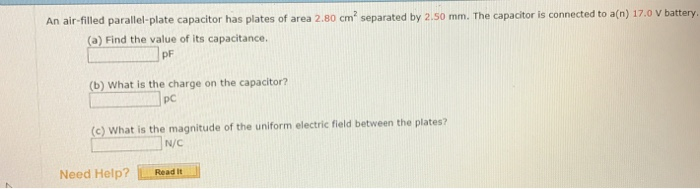An air-filled parallel-plate capacitor has plates of area 2.80 cm^2 separated by 2.50 mm. The capacitor is connected to a(n) 17.0 V battery. Find the value of its capacitance. _____ pF What is the charge on the capacitor? ______ pC What is the magnitude of the uniform electric field between the plates ___________ N/C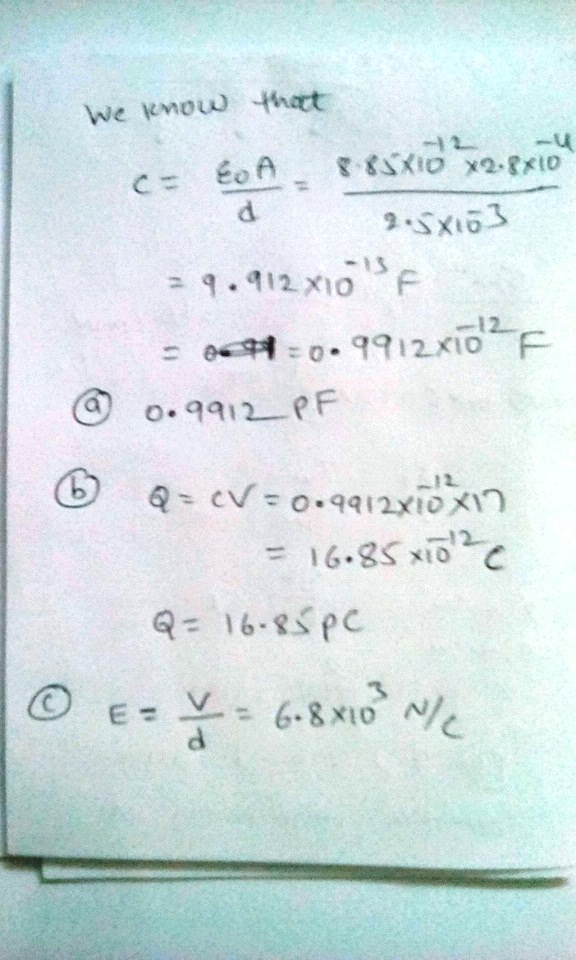Earn Coins

Coins can be redeemed for fabulous gifts.

Similar Homework Help Questions
• parallel-plate capacitor

An air-filled parallel-plate capacitor has plates of area 2.70 cm2 separated by 1.00 mm. The capacitor is connected to a 15.0 V battery.(a) Find the value of its capacitance.pF(b) What is the charge on the capacitor?pC(c) What is the magnitude of the uniform electric field between the plates?N/C

• I need the work for these to compare and check mine with! Problem: An air filled parallel plate capacitor has plates of area 2.3 cm separated by 1.5 mm. The capacitor is connected to a 12 V battery 3...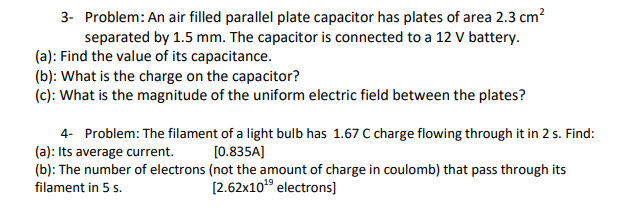I need the work for these to compare and check mine with! Problem: An air filled parallel plate capacitor has plates of area 2.3 cm separated by 1.5 mm. The capacitor is connected to a 12 V battery 3- (a): Find the value of its capacitance (b): What is the charge on the capacitor? (c): What is the magnitude of the uniform electric field between the plates? 4- Problem: The filament of a light bulb has 1.67 C charge flowing...

• Solve for a and b and show work please. An air-filled parallel-plate capacitor has plates of...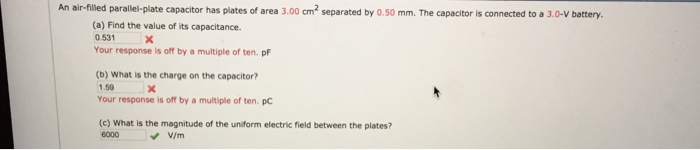Solve for a and b and show work please. An air-filled parallel-plate capacitor has plates of area 3.00 cm2 separated by 0.50 mm. The capacitor is connected to a 3.0-V battery. (a) Find the value of its capacitance. 0.531 Your response is off by a multiple of ten. p (b) What is the charge on the capacitor? 1.59 Your response is off by a multiple of ten. pc (c) What is the magnitude of the uniform electric field between the...

• An air-filled capacitor consists of two parallel plates, each with an area of 7.60 cm2, separated...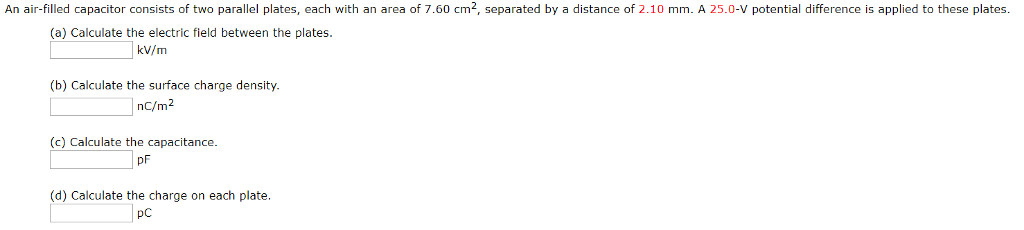An air-filled capacitor consists of two parallel plates, each with an area of 7.60 cm2, separated by a distance of 2.10 mm. A 25.0-V potential difference is applied to these plates (a) Calculate the electric field between the plates kV/m (b) Calculate the surface charge density. nc/m2 (c) Calculate the capacitance. pF (d) Calculate the charge on each plate pc

• An air-filled capacitor consists of two parallel plates, each with an area of 7.60 cm2, separated...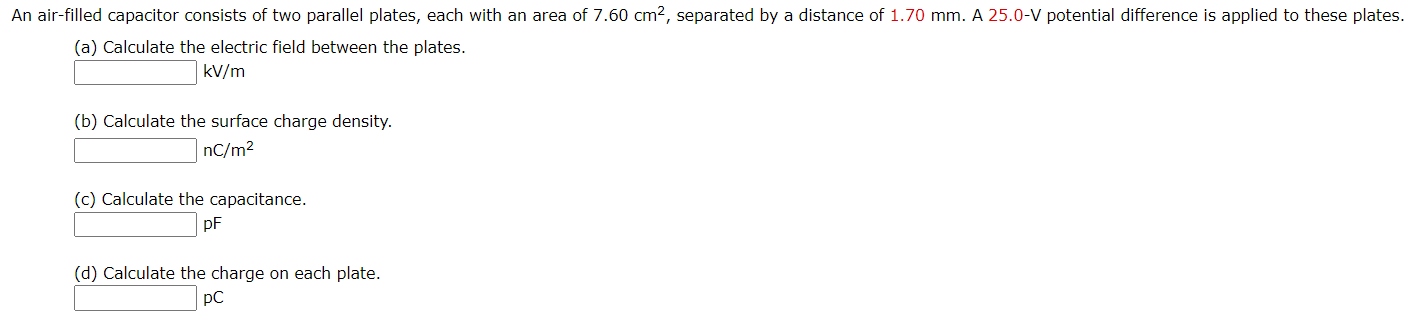An air-filled capacitor consists of two parallel plates, each with an area of 7.60 cm2, separated by a distance of 1.70 mm. A 25.0-V potential difference is applied to these plates. (a) Calculate the electric field between the plates. kV/m (b) Calculate the surface charge density. nC/m2 (c) Calculate the capacitance. pF (d) Calculate the charge on each plate. pC

• An air-filled, parallel plate capacitor has plates of area A = .006 [m^2] and are separated...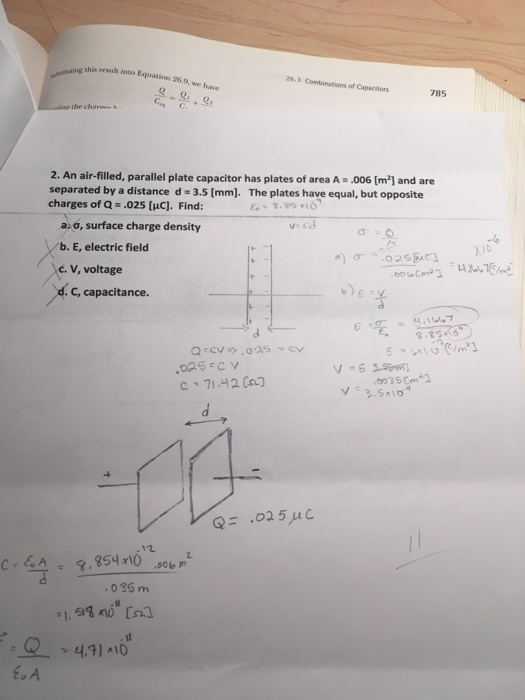An air-filled, parallel plate capacitor has plates of area A = .006 [m^2] and are separated by a distance d = 3.5 [mm]. The plates have equal, but opposite charges of Q = .025 [mu C]. Find: sigma, surface charge density E, electric field V, voltage C, capacitance.

• Parallel plate capacitor problem....

An air-filled parallel plate capacitor has plates of area 2.30 cm^2 seperated by 1.50 mm. The capacitor is connected to a 12.0 v battery.a) find the value of its capacitance.b) what is the charge on the capacitor?c) what is the magnitude of the uniform electric field between the plates?Thanks

• A parallel-plate air-filled capacitor having area 34 cm^2 and plate spacing 1.2 mm is charged to...

A parallel-plate air-filled capacitor having area 34 cm^2 and plate spacing 1.2 mm is charged to a potential difference of 700 V. Find (a) the capacitance, (b) the magnitude of the charge on each plate, (c) the stored energy, (d) the electric field between the plates, (e) the energy density between the plates.

• A parallel-plate air-filled capacitor having area 31 cm2 and plate spacing 3.0 mm is charged to...

A parallel-plate air-filled capacitor having area 31 cm2 and plate spacing 3.0 mm is charged to a potential difference of 400 V. Find the following values. (a) the capacitance pF (b) the magnitude of the charge on each plate nC (c) the stored energy μJ (d) the electric field between the plates V/m (e) the energy density between the plates J/m3

• A parallel-plate air-filled capacitor having area 44 cm2 and plate spacing 5.0 mm is charged to...

A parallel-plate air-filled capacitor having area 44 cm2 and plate spacing 5.0 mm is charged to a potential difference of 850 V. Find the following values. (a) the capacitance (pF) (b) the magnitude of the charge on each plate (nC) (c) the stored energy (μJ) (d) the electric field between the plates (V/m) (e) the energy density between the plates (J/m3)